Search this API

## y.layout Class SliderEdgeLabelModel

```java.lang.Objecty.layout.SliderEdgeLabelModel
```
All Implemented Interfaces:
EdgeLabelModel

`public class SliderEdgeLabelModelextends java.lang.Objectimplements EdgeLabelModel`

`SliderEdgeLabelModel` allows placement of labels at a set of continuous positions along both sides of an edge or directly on the edge path.

The set of positions can be influenced by specifying the `density value` which controls the spacing between adjacent label positions.

Furthermore, it's possible to specify distance values that control the distance between label and edge and between label and nodes.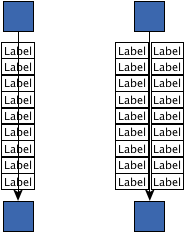`CENTER_SLIDER` mode on the left and `SIDE_SLIDER` mode on the right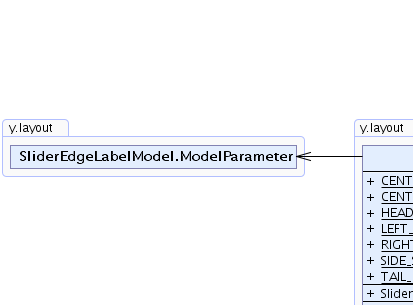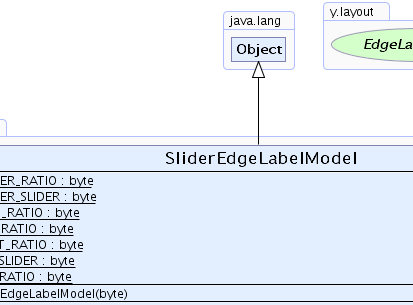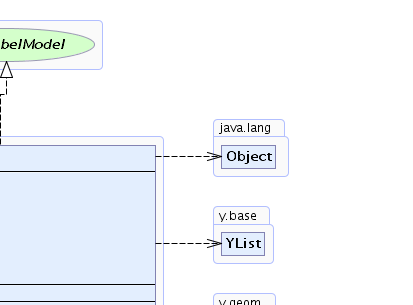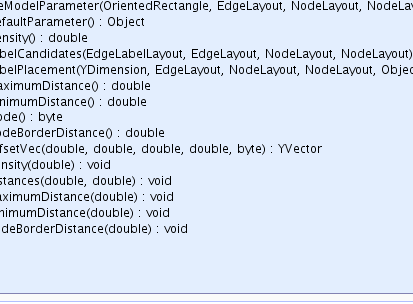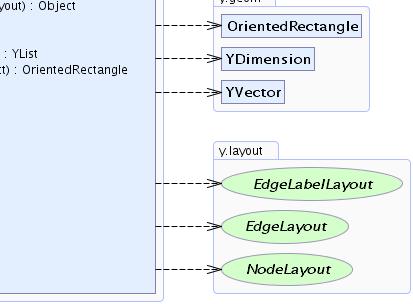Nested Class Summary
`static class` `SliderEdgeLabelModel.ModelParameter`
Encodes the model parameters for the two slider modes.

Field Summary
`static byte` `CENTER_RATIO`
Ratio specifier that describes a label placement on the edge's path.
`static byte` `CENTER_SLIDER`
Slider mode specifier which describes continuous label positions directly on the edge path.
`static byte` `HEAD_RATIO`
Ratio specifier that describes a label placement left or above of the edge's path.
`static byte` `LEFT_RATIO`
Ratio specifier that describes a label placement left of the edge's path.
`static byte` `RIGHT_RATIO`
Ratio specifier that describes a label placement right of the edge's path.
`static byte` `SIDE_SLIDER`
Slider mode specifier which describes continuous label positions along the sides of the edge path.
`static byte` `TAIL_RATIO`
Ratio specifier that describes a label placement right or below of the edge's path.

Constructor Summary
`SliderEdgeLabelModel(byte mode)`
Creates a new instance of `SliderEdgeLabelModel` with the given mode.

Method Summary
` java.lang.Object` ```createModelParameter(OrientedRectangle labelBounds, EdgeLayout edgeLayout, NodeLayout sourceNode, NodeLayout targetNode)```
Creates a model parameter that represents the given edge label position within this model.
` java.lang.Object` `getDefaultParameter()`
Returns a model parameter that encodes the default position of this model's allowed edge label positions.
` double` `getDensity()`
Returns the density for the generation of label candidate positions.
` YList` ```getLabelCandidates(EdgeLabelLayout labelLayout, EdgeLayout edgeLayout, NodeLayout sourceLayout, NodeLayout targetLayout)```
Returns all `EdgeLabelCandidate`s that describe valid label positions within this model.
` OrientedRectangle` ```getLabelPlacement(YDimension labelSize, EdgeLayout edgeLayout, NodeLayout sourceNode, NodeLayout targetNode, java.lang.Object para)```
Returns the oriented box of the label for the position encoded by the given model parameter.
` double` `getMaximumDistance()`
Returns the maximum distance between the label's bounding box and the edge's path.
` double` `getMinimumDistance()`
Returns the minimum distance between the label's bounding box and the edge's path.
` byte` `getMode()`
Returns the model's slider mode which determines whether the label slides `on the edge` or `beside the edge`.
` double` `getNodeBorderDistance()`
Returns the minimum distance between the label's bounding box and a node's borders.
` YVector` ```getOffsetVec(double dx, double dy, double width, double height, byte position)```
Returns the offset vector for a given edge label and a given edge segment.
` void` `setDensity(double density)`
Specifies the density for the generation of label candidate positions.
` void` ```setDistances(double minDistance, double maxDistance)```
Specifies the minimum and maximum distances between the label's bounding box and the edge's path.
` void` `setMaximumDistance(double distance)`
Specifies the maximum distance between the label's bounding box and the edge's path.
` void` `setMinimumDistance(double distance)`
Specifies the minimum distance between the label's bounding box and the edge's path.
` void` `setNodeBorderDistance(double value)`
Specifies the minimum distance between the label's bounding box and a node's borders.

Methods inherited from class java.lang.Object
`clone, equals, finalize, getClass, hashCode, notify, notifyAll, toString, wait, wait, wait`

Field Detail

### CENTER_SLIDER

`public static final byte CENTER_SLIDER`
Slider mode specifier which describes continuous label positions directly on the edge path.

`getMode()`, Constant Field Values
Sample Graph: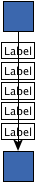Labels are placed centered on the edge

### SIDE_SLIDER

`public static final byte SIDE_SLIDER`
Slider mode specifier which describes continuous label positions along the sides of the edge path.

`getMode()`, Constant Field Values
Sample Graph:Labels are placed beside the edge

### CENTER_RATIO

`public static final byte CENTER_RATIO`
Ratio specifier that describes a label placement on the edge's path.

This ratio will only by considered if the label model's mode is `CENTER_SLIDER`.
`getMode()`, Constant Field Values
Sample Graph: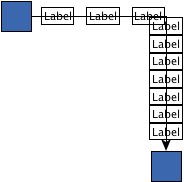Labels are placed on the edge

### TAIL_RATIO

`public static final byte TAIL_RATIO`
Ratio specifier that describes a label placement right or below of the edge's path.

This ratio will only by considered if the label model's mode is `SIDE_SLIDER`.
`getMode()`, Constant Field Values
Sample Graph:Labels are placed right or below of the edge

`public static final byte HEAD_RATIO`
Ratio specifier that describes a label placement left or above of the edge's path.

This ratio will only by considered if the label model's mode is `SIDE_SLIDER`.
`getMode()`, Constant Field Values
Sample Graph:Labels are placed left or above of the edge

### LEFT_RATIO

`public static final byte LEFT_RATIO`
Ratio specifier that describes a label placement left of the edge's path.

This ratio will only by considered if the label model's mode is `SIDE_SLIDER`.
`getMode()`, Constant Field Values
Sample Graph:Labels are placed left of the edge

### RIGHT_RATIO

`public static final byte RIGHT_RATIO`
Ratio specifier that describes a label placement right of the edge's path.

This ratio will only by considered if the label model's mode is `SIDE_SLIDER`.
`getMode()`, Constant Field Values
Sample Graph:Labels are placed right of the edge
Constructor Detail

### SliderEdgeLabelModel

`public SliderEdgeLabelModel(byte mode)`
Creates a new instance of `SliderEdgeLabelModel` with the given mode.

Parameters:
`mode` - the slider mode
`getMode()`
Method Detail

### getMode

`public byte getMode()`
Returns the model's slider mode which determines whether the label slides `on the edge` or `beside the edge`.

Returns:
the model's slider mode
`CENTER_SLIDER`, `SIDE_SLIDER`

### setDistances

```public void setDistances(double minDistance,
double maxDistance)```
Specifies the minimum and maximum distances between the label's bounding box and the edge's path.

The distances need to be non-negative.

Parameters:
`minDistance` - the minimum distance between label and edge
`maxDistance` - the maximum distance between label and edge
`getMaximumDistance()`, `getMinimumDistance()`, `setMaximumDistance(double)`, `setMinimumDistance(double)`

### getMaximumDistance

`public double getMaximumDistance()`
Returns the maximum distance between the label's bounding box and the edge's path.

The distance needs to be non-negative.

Returns:
the maximum distance between label and edge
`setMaximumDistance(double)`, `setDistances(double, double)`

### setMaximumDistance

`public void setMaximumDistance(double distance)`
Specifies the maximum distance between the label's bounding box and the edge's path.

The distance needs to be non-negative.

Default Value:
The default value is 1.0.
Parameters:
`distance` - the maximum distance between label and edge
`setDistances(double, double)`

### getMinimumDistance

`public double getMinimumDistance()`
Returns the minimum distance between the label's bounding box and the edge's path.

The distance needs to be non-negative.

Returns:
the minimum distance between label and edge
`setMinimumDistance(double)`, `setDistances(double, double)`

### setMinimumDistance

`public void setMinimumDistance(double distance)`
Specifies the minimum distance between the label's bounding box and the edge's path.

The distance needs to be non-negative.

Default Value:
The default value is 1.0.
Parameters:
`distance` - the minimum distance between label and edge
`setDistances(double, double)`

### getDensity

`public double getDensity()`
Returns the density for the generation of label candidate positions.

The density is defined as a value between `0` and `1`. Lower densities will result in less `LabelCandidate`s. A density value of `1.0` generates the maximum number of possible candidate positions without overlap.

Returns:
the density value
`setDensity(double)`

### setDensity

`public void setDensity(double density)`
Specifies the density for the generation of label candidate positions.

The density is defined as a value between `0` and `1`. Lower densities will result in less `LabelCandidate`s. A density value of `1.0` generates the maximum number of possible candidate positions without overlap.

Default Value:
The default value is 1.0.
Parameters:
`density` - the density value
Throws:
`java.lang.IllegalArgumentException` - if the specified density is less than `0` or greater than `1`
Sample Graphs: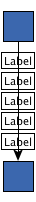`1`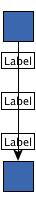`0.5`

### getNodeBorderDistance

`public double getNodeBorderDistance()`
Returns the minimum distance between the label's bounding box and a node's borders.

The distance must be non-negative.

Returns:
the distance between label and node border
`setNodeBorderDistance(double)`

### setNodeBorderDistance

`public void setNodeBorderDistance(double value)`
Specifies the minimum distance between the label's bounding box and a node's borders.

The distance must be non-negative.

Default Value:
The default value is 9.125.
Parameters:
`value` - the distance between label and node border
Sample Graphs:`9.125`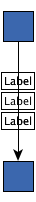`20`

### getDefaultParameter

`public java.lang.Object getDefaultParameter()`
Returns a model parameter that encodes the default position of this model's allowed edge label positions.

This parameter can be passed to `getLabelPlacement(YDimension, EdgeLayout, NodeLayout, NodeLayout, Object)` to retrieve the corresponding label box.

The default positions for both `slider modes` are relative to the first edge segment, either at the beginning of the segment (`SIDE_SLIDER`) or at its middle (`CENTER_SLIDER`).

Specified by:
`getDefaultParameter` in interface `EdgeLabelModel`
Returns:
the model parameter describing the default placement of the edge label
`getMode()`

### getLabelPlacement

```public OrientedRectangle getLabelPlacement(YDimension labelSize,
EdgeLayout edgeLayout,
NodeLayout sourceNode,
NodeLayout targetNode,
java.lang.Object para)```
Description copied from interface: `EdgeLabelModel`
Returns the oriented box of the label for the position encoded by the given model parameter.

Specified by:
`getLabelPlacement` in interface `EdgeLabelModel`
Parameters:
`labelSize` - the width and height of the label
`edgeLayout` - the layout of the edge to which the label belongs
`sourceNode` - the layout of the source node of the label-owning edge
`targetNode` - the layout of the target node of the label-owning edge
`para` - the model parameter that describes the abstract position of the label within this model
Returns:
the oriented bounds of the label

### getLabelCandidates

```public YList getLabelCandidates(EdgeLabelLayout labelLayout,
EdgeLayout edgeLayout,
NodeLayout sourceLayout,
NodeLayout targetLayout)```
Returns all `EdgeLabelCandidate`s that describe valid label positions within this model. The number of candidates and their respective locations are computed depending on the geometries of both label and edge.

Specified by:
`getLabelCandidates` in interface `EdgeLabelModel`
Parameters:
`labelLayout` - the label for which candidates should be generated
`edgeLayout` - the layout of the edge to which the label belongs
`sourceLayout` - the layout of the source node of the label-owning edge
`targetLayout` - the layout of the target node of the label-owning edge
Returns:
a list of `EdgeLabelCandidate` instances

### createModelParameter

```public java.lang.Object createModelParameter(OrientedRectangle labelBounds,
EdgeLayout edgeLayout,
NodeLayout sourceNode,
NodeLayout targetNode)```
Description copied from interface: `EdgeLabelModel`
Creates a model parameter that represents the given edge label position within this model.

The created model parameter is the closest parameter representation of the given label location that can be achieved within this model.

This parameter can be passed to `EdgeLabelModel.getLabelPlacement(YDimension, EdgeLayout, NodeLayout, NodeLayout, Object)` to retrieve the current label box.

A model parameter can be an arbitrary `Object`. However it must contain all information to allow restoring of the encoded location with this `EdgeLabelModel`.

Specified by:
`createModelParameter` in interface `EdgeLabelModel`
Parameters:
`labelBounds` - the oriented box of the label, encoding the label location for which the parameter should be created
`edgeLayout` - the layout of the edge to which the label belongs
`sourceNode` - the layout of the source node of the label-owning edge
`targetNode` - the layout of the target node of the label-owning edge
Returns:
the model parameter representing the given label location
`EdgeLabelModel.getLabelPlacement(YDimension, EdgeLayout, NodeLayout, NodeLayout, Object)`

### getOffsetVec

```public YVector getOffsetVec(double dx,
double dy,
double width,
double height,
byte position)```
Returns the offset vector for a given edge label and a given edge segment. The offset vector describes where to move a label candidate such that it keeps a certain distance to the edge's path.

Parameters:
`dx` - the x-coordinate's delta for an edge segment
`dy` - the y-coordinate's delta for an edge segment
`width` - the label's width
`height` - the label's height
`position` - one of the valid ratio specifiers
Returns:
the offset vector